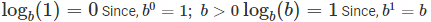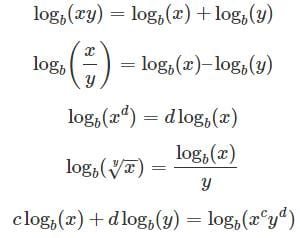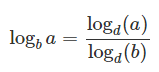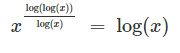Logarithm Formula

# Logarithm Formula - Commerce

 1 Crore+ students have signed up on EduRev. Have you?

Logarithms are the opposite phenomena of exponential like subtraction is the inverse of addition process, and division is the opposite phenomena of multiplication. Logs “undo” exponentials.

Trivial IdentitiesBasic Logarithm FormulasChanging the Base#### ExponentsThe document Logarithm Formula - Commerce is a part of Commerce category.
All you need of Commerce at this link: Commerce

Track your progress, build streaks, highlight & save important lessons and more!(Scan QR code)

,

,

,

,

,

,

,

,

,

,

,

,

,

,

,

,

,

,

,

,

,

;